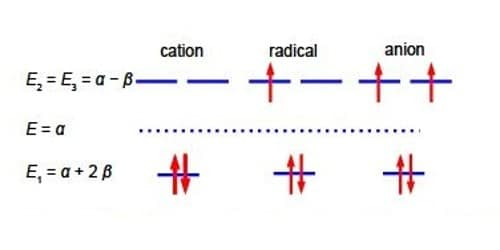# Sample Question: HMO Theory for Cyclopropenyl Cation, Radical and Anion

Sample Question: HMO Theory for Cyclopropenyl Cation, Radical, and Anion.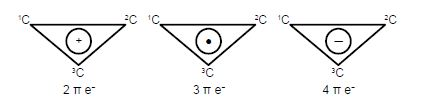(a) Write down the Hiickel secular determinant for the cyclic three C-atoms in terms of x, where x = (α – E)/β.

(b) Solve the secular determinant for x and then rewrite the solution in terms of (α – E)/β and so find the three allowed quantized energies (eigenvalues).

(c) Evaluate the normalized wavefunction (eigenvector) for each energy (eigenvalues).

(d) Which of the wavefunctions is the HOMO, LUMO or SOMO as appropriate, for each of the cyclopropenyl cation, radical and anion molecules?

Solution

It is the simplest molecular orbital theory of π-conjugated molecular systems. The cyclic species differs from the linear three-carbon species in that each ring C-atom is bonded to two other C-atoms. Thus by inspection, put x (α – E)/β  on the diagonal elements and 1 for the elements representing connected atoms in the secular determinant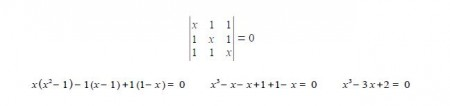This is a cubic equation and by inspection x = +1 is a root of the cubic, hence (x-1) is a factor of the cubic equation. We may find the other factor, which should be quadratic, by long division and then find the roots of the quadratic using the quadratic formula.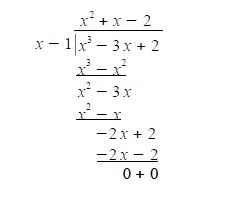The quadratic factor (x2+x-2) = 0 is solved as (x-1)(x+2) = 0 or if you prefer by using the quadratic formula. The Cyclopropenyl Cation molecules each have three eigenvalues of which two are identical (degenerate) and in increasing order of energy they are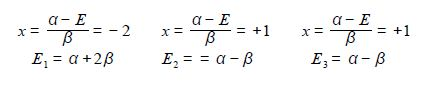The eigenvectors (wavefunctions) are obtained by solving the Hiickel matrix for Cyclopropenyl Cation molecules. For E1 = α +2β, then (α – E1) = -2β,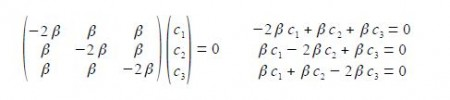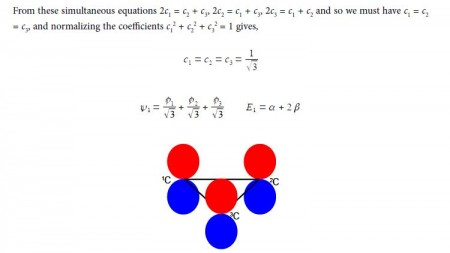In the above Figure, we see that the HMO ψ1 is a delocalized it-bond involving all three C-atoms. The degenerate wavefunctions ψ2 and ψ3 are obtained from solving the Hiickel matrix for E2 = (α – β) and also E3 = (α — β) so for (α – E2) = β and (α — E3) = β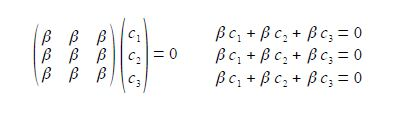The two degenerate MOs do not give independent solutions for the coefficients and we are free to choose the first solution as long as it satisfies the secular equation c1 + c2 + c3 = 0 above. For ψ2 we can choose that c3 = 0 and then c, = – c2 after normalizing the coefficients c12 + c22 + c32 = 1 we have c1 = —c2 = 1/√2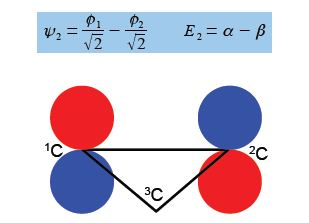For ψ2 we chose that c1 = – c2 then the other degenerate wavefunction ψ3 must have c1 = +c2 so substituting this into the secular equation c1 + c2 + c3 = 0 gives c3 = – 2c1 = -2c2. After normalizing the coefficients c12 + c22 + c32 = 1 we have the ψ3 wavefunction with c1 = c2 = 1/√6 and c3 = —2/√6 and so,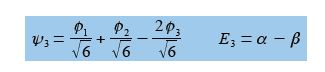Below Figure shows the MO energy diagrams for the three Cyclopropenyl Cation molecules.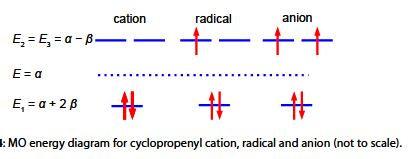The Cyclopropenyl Cation with two 2p electrons with n-electron energy of (2α + 4β) which compared with two isolated 2p electrons of energy 2α has stabilization energy of (2α + 4β) – 2α = 4β. The cyclopropenyl cation possesses a ring current. As we will see it is the most stable of the three molecules also its π-electrons are delocalized (aromatic) agreeing with Hiidcers (4n+2) rule for monocytic compounds. The stabilization of aromaticity is weakened by the extreme ring strain.

The cydopropenyl radical with three 2p electrons with a π-electrons energy of 2(π + 2β) + (α -β) = (3α + 3β) which compared with three isolated 2p electrons of energy 3a has a stabilization energy of (3α +3β) – 3α = 3β.

The cydopropenyl anion with four 2p electrons with with a π-electron energy of 2(α + 2β) + 2(α – β) = (4α + 2β) which compared with four isolated 2p electrons of energy 4α has a stabilization energy of only (4α + 2β) – 4α = 2β. It is also a diradical in order to satisfy Hund’s rule of maximum multiplicity.

[HMO Theory uses the following approximations: π-electron approximation; LCAO representation of the π-molecular orbitals; neglect of electron-electron and nuclear-nuclear repulsions.]